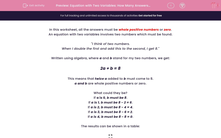# Equation with Two Variables: How Many Answers? (2)

In this worksheet, students must find how many integer solutions there are to a given equation with two variables.This content is premium and exclusive to EdPlace subscribers.Key stage:  KS 2

Curriculum topic:   Maths and Numerical Reasoning

Curriculum subtopic:   Equations and Algebra

Difficulty level:#### Worksheet Overview

In this worksheet, all the answers must be whole positive numbers or zero.

An equation with two variables involves two numbers which must be found.

"I think of two numbers.

When I double the first and add this to the second, I get 8."

Written using algebra, where a and b stand for my two numbers, we get:

2a + b = 8

This means that twice a added to b must come to 8.

a and b are whole positive numbers or zero.

What could they be?

If a is 0, b must be 8.

If a is 1, b must be 8 - 2 = 6.

If a is 2, b must be 8 - 4 = 4.

If a is 3, b must be 8 - 6 = 2.

If a is 4, b must be 8 - 8 = 0.

The results can be shown in a table:

a   b
0 8
1 6
2 4
3 2
4 0

There are 5 solutions.

### What is EdPlace?

We're your National Curriculum aligned online education content provider helping each child succeed in English, maths and science from year 1 to GCSE. With an EdPlace account you’ll be able to track and measure progress, helping each child achieve their best. We build confidence and attainment by personalising each child’s learning at a level that suits them.

Get started